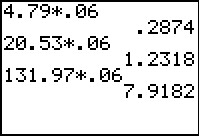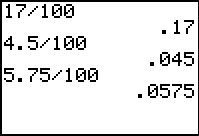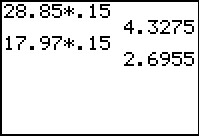# Activities

••• ##### Subject Area

• Math: Algebra I: Linear Functions

• ##### Author6-8
9-12

15 Minutes

• ##### Device
• TI-83 Plus Family
• TI-84 Plus
• TI-84 Plus Silver Edition
•TI-84 Plus C Silver Edition
•TI-84 Plus CE

## Taxes & Tips#### Activity Overview

Students explore the taxes and tips percentages commonly used in stores and restaurants. They will first develop the pattern of converting a percent to a decimal.

#### Key Steps

•In this activity, students will explore the taxes and tips percentages commonly used in stores and restaurants. They will first develop the pattern of converting a percent to a decimal.

•Then students will use a tax equation model to calculate the tax on several items. They will compare the difference of calculating the taxes on individual items, then summing to the calculating the sum of the item prices multiplied by the tax rate.

•Students will finally use mental math estimation to calculate 15% or 20% of a restaurant bill or calculate the tax on a high priced item. They will compare their estimation to the actual answer.